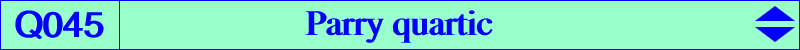X(2), X(8), X(369), X(519) Ga, Gb, Gc : vertices of the antimedial triangle Ma, Mb, Mc : midpoints of ABC infinite points of BC, CA, AB remark : X(369) and X(3232) are the 1st and 2nd trisected perimeter pointsIn memoriam Cyril Parry who died on February, 13 2005 Q045 is the locus of point M such that X(8), M, the centroid of the cevian triangle of M are collinear. See the related Q033. Q045 is an example of curve passing through X(369), the 1st trisected perimeter point. Its equation is remarkably simple. Q045 is a quartic with four real asymptotes : three are parallel to the sidelines of ABC and the last one is parallel to the line IG. The tangents at A, B, C, Ga, Gb, Gc, G concur at X(8), the Nagel point. More generally, the polar line (with respect to the quartic) of any point on pK(X2, X7) passes through X(8). See also K311 = pK(X2, X320), a cubic through X(369) and X(3232) and also Table 42 for other curves passing through X(369). A construction is given in the page Q002. Other locus properties : Q045 is the locus of point M such that • X(8), M, tM/M are collinear where tM/M is the cevian quotient of the isotomic conjugate tM of M and M. • M, tM, X7/tM are collinear.